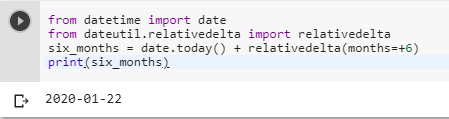2 views
in Python

I am using the datetime Python module. I am looking to calculate the date 6 months from the current date. Could someone give me a little help doing this?

The reason I want to generate a date of 6 months from the current date is to produce a review date. If the user enters data into the system it will have a review date of 6 months from the date they entered the data.

by (106k points)

To calculate the date six months from the current date you can do by using the datetime Python module below is the code for the same:-

from datetime import date

from dateutil.relativedelta import relativedelta

six_months = date.today() + relativedelta(months=+6)

print(six_months)Another thing you can do is to get the date after six months from the current date is by using the following method:-

import datetime

print (datetime.date.today() + datetime.timedelta(6*365/12)).isoformat()